# Standard Order

## Smallest

6.7 × 103 = 6700

6.27 × 103 = 6270

6.7 × 104 = 67000

6.2 × 104 = 62000

6.2 × 103 = 6200

6.07 × 104 = 60700

6.77 × 105 = 677000

6.2 × 105 = 620000

6.72 × 105 = 672000

6.07 × 103 = 6070

## Largest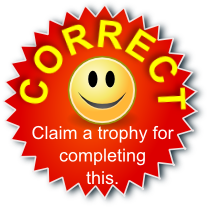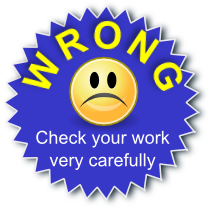There are more activities on the more general topic of Indices and some particularly useful links can be found below:

#### Standard Form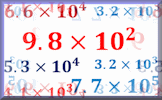A self marking exercise on converting standard form numbers to ordinary numbers and vica-versa.

Transum.org/go/?to=standardform

#### Indices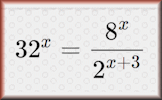A self marking exercise on indices (powers) including evaluating statements and solving equations.

Transum.org/go/?to=powers

#### Indices Pairs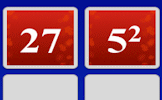The traditional pairs or pelmanism game adapted to test knowledge of indices. Enjoy the game!

Transum.org/go/?to=indices

The solutions to this and other Transum puzzles, exercises and activities are available here when you are signed in to your Transum subscription account. If you do not yet have an account and you are a teacher, tutor or parent you can apply for one here.

Do you have any comments? It is always useful to receive feedback and helps make this free resource even more useful for those learning Mathematics anywhere in the world. Click here to enter your comments.

## Description of Levels

CloseLevel 1 - Five numbers to sort, all with positive powers of 10

Level 2 - Ten numbers to sort, all with positive powers of 10

Level 3 - Ten numbers to sort, all with negative powers of 10

Level 4 - Ten mixed numbers to sort, all greater than 1

Level 5 - Ten mixed numbers to sort, all less than 1For All: